# VBA – Pivot Table Filter

This tutorial will demonstrate how to use the Pivot Table Filter in VBA.

Pivot tables are an exceptionally powerful data tool of Excel. Pivot tables enable us to analyze and interpret large amounts of data by grouping and summarizing fields and rows. We can apply filters to our pivot tables to enable us to quickly see the data that is relevant to us.

Firstly, we need to create a Pivot table for our data.  (Click Here for our VBA Pivot Table Guide)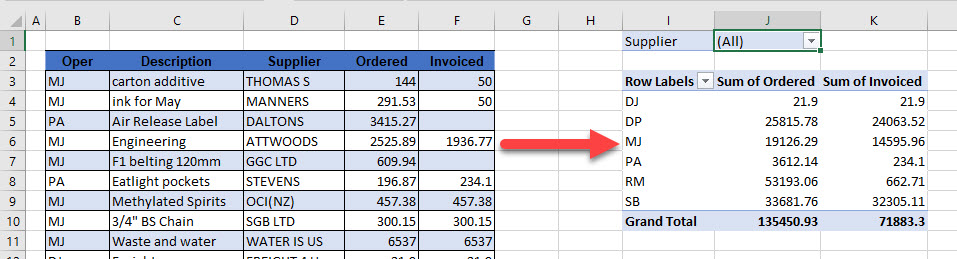## Creating a Filter Based on a Cell Value

In this example we will filter a Pivot Table based on a cell value.

In an empty cell to the right of the Pivot table, create a cell to hold the filter, and then type the data into the cell that you wish to filter the Pivot table on.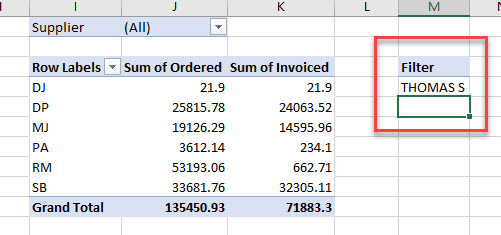Create the following VBA Macro:

``````Sub FilterPageValue()
Dim pvFld As PivotField
Dim strFilter As String
Set pvFld = ActiveSheet.PivotTables("PivotTable1").PivotFields("Supplier")
strFilter = ActiveWorkbook.Sheets("Sheet1").Range("M4").Value
pvFld.CurrentPage = strFilter
End Sub``````

Run the macro to apply the filter.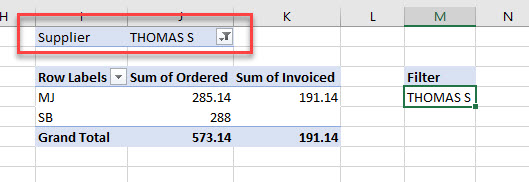To clear the filter, create the following macro:

``````Sub ClearFilter()
Dim pTbl As PivotTable
Set pTbl = ActiveSheet.PivotTables("PivotTable1")
pTbl.ClearAllFilters
End Sub``````

The filter will then be removed.

We can then amend the filter criteria to filter on a row in the Pivot table rather than the Current Page.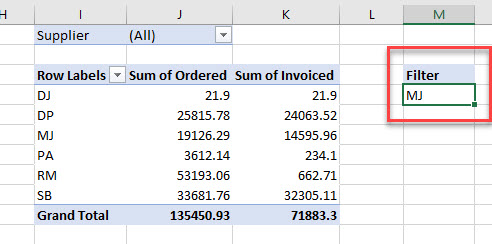Typing the following macro will then enable us to filter on the Row (note that the Pivot Field to filter on is now the Oper rather than the Supplier).

``````Sub FilterRowValue()
Dim pvFld As PivotField
Dim strFilter As String
Set pvFld = ActiveSheet.PivotTables("PivotTable1").PivotFields("Oper")
strFilter = ActiveWorkbook.Sheets("Sheet1").Range("M4").Value
End Sub``````

Run the macro to apply the filter.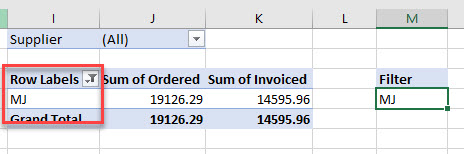### Using Multiple Criteria in a Pivot Filter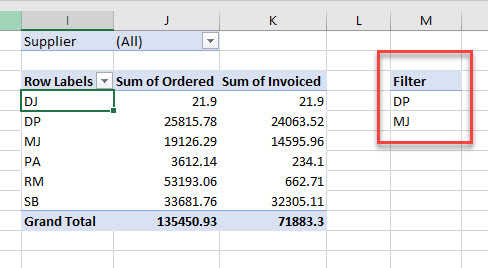However, as the standard filter hides the rows that are not required, we need to loop through the criteria and show the ones that are requited, while hiding the ones that are not required. This is done by creating an Array variable and using a couple of Loops in the code.

``````Sub FilterMultipleRowItems()
Dim vArray As Variant
Dim i As Integer, j As Integer
Dim pvFld As PivotField
Set pvFld = ActiveSheet.PivotTables("PivotTable1").PivotFields("Oper")
vArray = Range("M4:M5")
pvFld.ClearAllFilters

With pvFld
For i = 1 To pvFld.PivotItems.Count
j = 1
Do While j <= UBound(vArray, 1) - LBound(vArray, 1) + 1
If pvFld.PivotItems(i).Name = vArray(j, 1) Then
pvFld.PivotItems(pvFld.PivotItems(i).Name).Visible = True
Exit Do
Else
pvFld.PivotItems(pvFld.PivotItems(i).Name).Visible = False
End If
j = j + 1
Loop
Next i
End With
End Sub``````

## Creating a Filter Based on a Variable

We can use the same concepts to create filters based on variables in our code rather than the value in a  cell. This time, the filter variable (strFilter) is populated in the code itself (eg: Hard-coded into the macro).

``````Sub FilterTextValue()
Dim pvFld As PivotField
Dim strFilter As String
Set pvFld = ActiveSheet.PivotTables("PivotTable1").PivotFields("Supplier")
strFilter = "THOMAS S"
pvFld.CurrentPage = strFilter
End Sub``````

Stop searching for VBA code online. Learn more about AutoMacro - A VBA Code Builder that allows beginners to code procedures from scratch with minimal coding knowledge and with many time-saving features for all users!